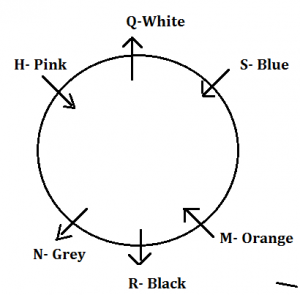Latest Banking jobs   »

# Reasoning Ability Quiz For FCI Phase I 2023- 11th January

Directions (1-5): Study the following information carefully and answer the given questions.
Six friends H, M, N, Q, R and S are sitting around the circular table but not necessarily in the same order. Some of them are facing outside the center while some of them are facing towards the center. Each of them likes different color viz. White, Black, Pink, Blue, Orange and Grey but not necessarily in the same order.
S sits second to the left of R. N sits opposite to the one who likes Blue. Q faces outside the center and doesn’t like Black. H doesn’t like White and facing opposite direction to N. H sits immediate right of N, who doesn’t like Blue. Two people sits between H and the one who likes Orange. The one who likes White is not an immediate neighbor of R. The one who sits second to the right of Q likes Orange. The one who likes pink sits opposite to M. Neither S nor N likes White. The one who likes grey sits second to the left of the one who likes White. S faces same direction as M but opposite to Q.

Q1. Who among the following person sits to the immediate left of M?
(a) H
(b) R
(c) S
(d) Q
(e) N

Q2. Which of the following color is liked by R?
(a) Grey
(b) Black
(c) De-Milano
(d) Orange
(e) Blue

Q3. Which of the following combinations of “Person – Color” is true?
(a) N – Black
(b) S – Pink
(c) Q- Orange
(d) N – Grey
(e) R- Pink

Q4. How many persons sits between Q and R when counted in clock-wise direction from Q?
(a) Three
(b) Two
(c) One
(d) More than three
(e) None

Q5. Who among the following faces outside the center?
(a) S
(b) M
(c) N
(d) H
(e) None of these

Q6. If 2 is subtracted from each even number and 1 is added to each odd number in the number 75847361, then how many digits will appear twice in the new number thus formed?
(a) One
(b) Two
(c) Three
(d) Four
(e) More than four

Q7. There are five persons i.e., P, Q, R, S and T. If S is shorter than T and P. Q is taller than T who is not the taller than P. Q is taller than only two persons. P is not the tallest, then who is the third tallest person among all?
(a) P
(b) S
(c) Q
(d) R
(e) None of these

Q8. In a class Shivani is 20th from the top and Monica is 20th from the bottom. Dheeraj is at 6th rank below Shivani and at 5th rank above Monica. How many students are in the class if list includes all the students of the class?
(a) 50
(b) 51
(c) 52
(d) 58
(e) None of these

Q9. Dinesh rank is 6th from the top and eighteenth from the bottom in the class. How many students are there in the class?
(a) 29
(b) 28
(c) 27
(d) 25
(e) None of these

Q10. How many words can be formed from the 4th, 5th, 6th and 7th letter of a word ‘WITHDRAW’ by using each letter once in the word?
(a) More than three
(b) Three
(c) Two
(d) One
(e) None

Directions (11-15): In these questions, relationship between different elements is shown in the statements. These statements are followed by two conclusions. Mark answer
(a) if only conclusion I is true.
(b) if only conclusion II is true.
(c) if either conclusion I or II is true.
(d) if neither conclusion I nor II is true.
(e) if both conclusion I and II are true.

Q11. Statements: B≤C=D, A>B, D≤E, C≥F=G
Conclusions: I. B≥G
II. E≥G

Q12. Statements: H≥T, T>S≤Q, T≥U=V
Conclusions: I. U≤H
II. S<H

Q13. Statements: A>B≥L, R>B=H
Conclusions: I. A<L
II. R>L

Q14. Statements: P>K=L, P≤S<Q, T>K
Conclusions: I. Q>K
II. Q<T

Q15. Statements: P<H, V≥S>H, N≥V
Conclusions: I. N≥P
II. S>P

Solutions

Solutions (1-5):
Sol.S1. Ans. (b)
S2. Ans. (b)
S3. Ans. (d)
S4. Ans. (b)
S5. Ans. (c)

S6. Ans. (d)
Sol. The given number — 75847361
After applied operation– 86628442

S7. Ans. (c)
Sol. R > P > Q > T > S

S8. Ans. (a)
Sol. Total number of student in the class = 50

S9. Ans. (e)
Sol. Total number of students in the class= (6+18)-1= 23

S10. Ans. (d)
Sol. HARD

S11. Ans. (b)
Sol. I. B≥G (false)
II.E≥G (true)

S12. Ans. (e)
Sol. I. U≤H (true)
II.S<H (true)

S13. Ans. (b)
Sol. I. A<L (false)
II.R>L (true)

S14. Ans. (a)
Sol. I. Q>K (true)
II.Q<T (false)

S15. Ans. (b)
Sol. I. N≥P (false)
II.S>P (true)## FAQs

### What is the FCI Assistant Grade 3 Phase 1 Exam Date 2023?

The FCI Assistant Grade 3 Phase 1 Exam Dates 2023 are 1st, 7th, 14th & 21st January 2023.

#### Congratulations!Union Budget 2023-24: Free PDF